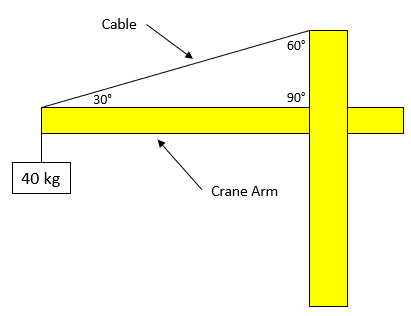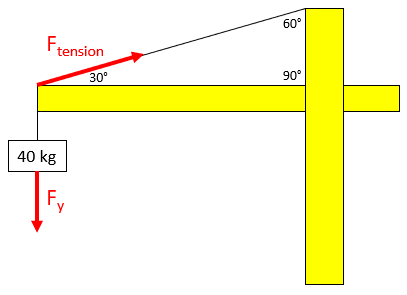## Construction Crane

Consider a construction crane in the below diagram is suspending a 40 kg payload. What is the tension in the diagonal cable that is supporting the load? Assume the crane arm is weightless.Hint
The problem is asking to solve for the y-component of $$F_{tension}$$ in the free body diagram below:Hint 2
To find $$F_y$$ , we can multiply the mass by gravity (Newton's 2nd law):
$$F_y=mass\times acceleration$$$The problem is asking to solve for the y-component of $$F_{tension}$$ in the free body diagram below:To find $$F_y$$ , we can multiply the mass by gravity (Newton's 2nd law): $$F_y=mass\times acceleration=40kg\times 9.8m/s^2=392\:N$$$
Because there is only one upward pulling cable in the vertical direction, it will counteract the entire downward force. Thus:
$$F_y=F_{tension}\cdot sin(30)=392N$$$$$F_{tension}=\frac{392N}{sin(30)}=\frac{392N}{0.5}=784\:N$$$
Despite the payload only causing a 392 N downward force, the cable has to work twice as hard to suspend the object due to its diagonal positioning.
784 N